Publication date: 11/10/2021

## Power for Two Independent Sample Means

Use the Power Explorer for Two Independent Sample Means to determine a sample size for a hypothesis test about the means from two groups. Select DOE > Sample Size Explorers > Power > Power for Two Independent Sample Means. Explore the trade offs between sample size, power, significance, and the hypothesized difference to detect. Sample size and power are associated with the following hypothesis test: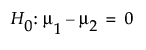versus the two-sided alternative: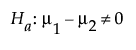or versus a one-sided alternative: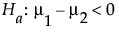or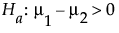where μ1 and μ2 are the true means of the two populations. It is assumed that the populations of interest are normally distributed and that you want to detect a difference of δ between the means.

### Power Explorer for Two Independent Sample Means Options

Set study assumptions and explore sample sizes using the radio buttons, text boxes, and sliders. The curve updates as you make changes to the settings. Alternatively, change settings by dragging the cross hairs on the curve or adjusting the values in the axis text boxes.

Test Type

Specifies a one or two-sided hypothesis test.

Fixed Parameters

Alpha

Specifies the probability of a type I error, which is the probability of rejecting the null hypothesis when it is true. It is commonly referred to as the significance level of the test. The default alpha level is 0.05.

Group 1 StdDev (σ1)

Specifies the assumed standard deviation for one of your groups, Group 1. An estimate of the error standard deviation could be the root mean square error (RMSE) from a previous model fit.

Group 2 StdDev (σ2)

Specifies the assumed standard deviation for the second group, Group 2. An estimate of the error standard deviation could be the root mean square error (RMSE) from a previous model fit.

Population standard deviations known

Specifies calculations based on a known, rather than assumed, population standard deviations.

Test Parameters

Difference to detect (Δ)

Specifies the smallest difference between the group means that you want to be able to declare statistically significant.

Group 1 Sample Size

Specifies the number of observations (runs, experimental units, or samples) needed for Group 1 in your experiment. Select Lock to lock the Group 1 sample size.

Group 2 Sample Size

Specifies the number of observations (runs, experimental units, or samples) needed for Group 2 in your experiment. Select Lock to lock the Group 2 sample size.

Total Sample Size

Specifies the total number of observations (runs, experimental units, or samples) needed for your experiment. The power curve is based on total sample size. Set Lock to lock the total sample size

Note: Adjusting the sample size for one group adjusts the total sample size unless the total sample size is locked. In that case, adjusting the sample size for one group adjust the sample size for the second group. Adjusting the total sample size adjusts the two groups proportionally, unless one group is locked. Use only one lock at a time.

Power

Specifies the probability of rejecting the null hypothesis when it is false. With all other parameters fixed, power increases as sample size increases.

Save Settings

Saves the current settings to the Saved Settings table. This enables you to save a set of alternative study plans. See Saved Settings in the Sample Size Explorers.

Make Data Collection Table

Creates a new data table that you can use for data collection.

### Statistical Details for Two Independent Means

The power calculations for testing the difference in means of two sample groups are based on the traditional t test, or if σ1 and σ2 are known, the z test.

For the case when σ is unknown and it is assumed that σ1=σ2=σ, the power is calculated based on the form of the alternative hypothesis. For a one-sided, higher alternative: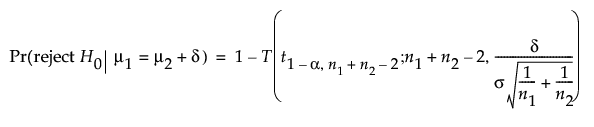For a one-sided, lower alternative: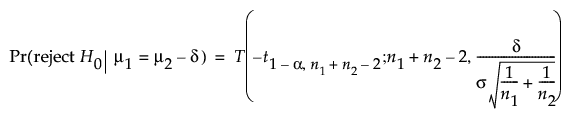For a two-sided alternative: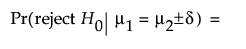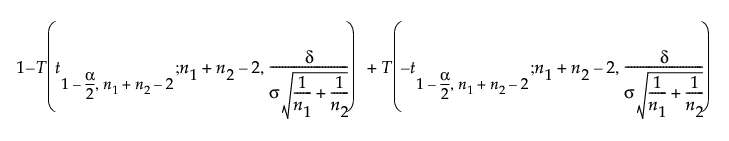where:

α is the significance level

n1 and n2 are the group sample sizes

σ is the pooled standard deviation

δ is the difference to detect

t1-α,ν is the (1 - α)th quantile of the central t-distribution with ν degrees of freedom

T(t; ν, λ) is the cumulative distribution function of the non-central t distribution with ν degrees of freedom and non-centrality parameter λ.

When σ1 and σ2 are not assumed to be equal then the degrees of freedom, n1+ n2 -2 in the above equations, is computed as follows: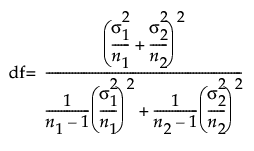When σ1 and σ2 are known the z distribution is used for the power calculation. The power is calculated based on the form of the alternative hypothesis. For a one-sided, higher alternative: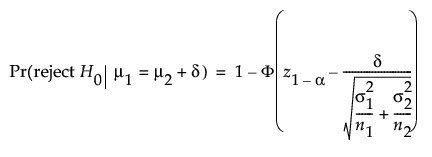For a one-sided, lower alternative: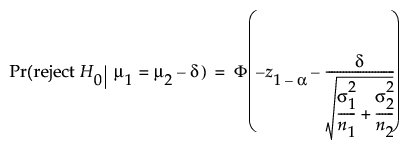For a two-sided alternative:where:

α is the significance level

n1 and n2 are the group sample sizes

σ1 and σ2 are known group standard deviations

δ is the difference to detect

z1-α is the (1 - α)th quantile of the z-distribution

Φ(x) is the cumulative distribution function of the normal distribution.